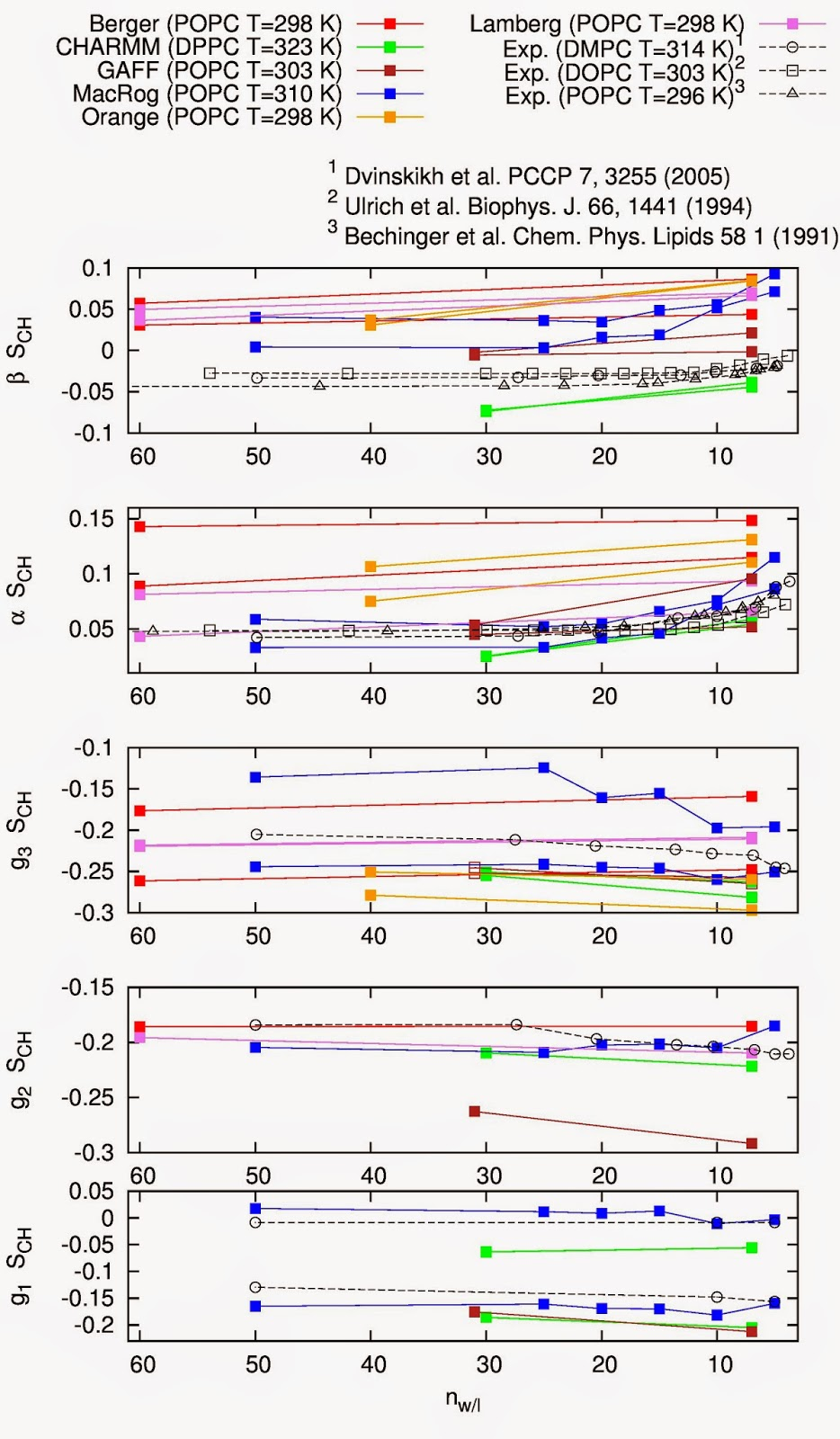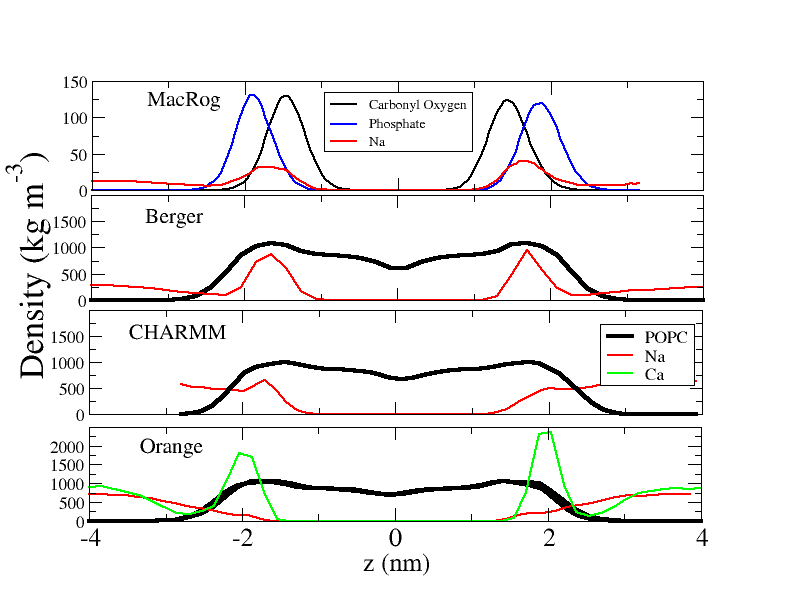## Friday, May 2, 2014

### Response of headgroup and glycerol order parameters to changing conditions: Results

During our project, the responses of headgroup and glycerol order parameters to hydration level, ion concentration and cholesterol content have already been reported for several models, and the results have been discussed to some extent in various comments. This post reviews the results for the responses reported in the nmrlipids-project so far.

Note on signs.
In the original manuscript and in the plots previously published in the blog (dehydration, NaCl, CaCl), the responses of the order parameters were discussed only in terms of their absolute values. From these plots the response of the $$\beta$$-carbon order parameter in all force fields except in CHARMM seemed to be qualitatively different than in experiments. Recently we have started to consider also the signs of the order parameters in addition to their magnitude. Even though the signs are not explicitly measured when responses are considered experimentally, it is reasonable to assume that the signs are not changing except for the case of adding a large amount of charges to the bilayer. Throughout this posting the signs of the order parameters are taken into account. For dehydration and ions the signs are relevant for the conclusions

The order parameters as a function of hydration level. It is clear from Fig. 1 that in experiments dehydration leads to more positive order parameters for the $$\beta$$ and $$\alpha$$ carbons. Results from all the experiments are in general very similar, despite some variation in lipid compositions and temperatures. The glycerol order parameters have been measured only in one experiment, where a decrease with dehydration was observed for the g$$_3$$ and g$$_2$$, while g$$_1$$ remains unchanged.Figure 1. Order parameters as a function of hydration level from the simulations reported through this blog and from the experiments by Dvinskikh et al. (2005), Ulrich et al. (1994) and Bechinger et al. (1991) In these experiments only absolute values were measured. The signs are assumed to be the same as in fully hydrated conditions measured by Hong et al. (1995), Hong et al. (1995) and Gross et al. (1997). Simulation values for some carbon segments are not seen since they are beyond the scale of the y-axis.

In simulations, as in experiments, the $$\beta$$- and $$\alpha$$-carbon order parameters increase with dehydration - even though the magnitudes are significantly different between different models and some models show significant forking. Note that this qualitative agreement between simulations and experiments for the $$\beta$$-carbon dehydration response differs from the conclusions of the original manuscript and of the discussions in the nmrlipids-blog. The reason for this is that previously only the absolute values have been discussed and many many force fields give wrong sign for the $$\beta$$-carbon order parameter: Increase in a negative order parameter decreases its absolute value (as seen in the experiments) while increase in a positive order parameter increases its absolute value (as seen in most simulations).

When the comparison between simulations and experiments is extended to the glycerol region, only the CHARMM36 force field can be interpreted to have all the order parameters quantitatively close to experiments, and to have qualitatively correct response to dehydration. However, the changes in the order parameters and difference between MacRog results and experiments are relatively small in the glycerol region.

The order parameters as a function of NaCl or CaCl$$_2$$ concentration. Fig. 2 shows the effect of NaCl on headgroup and glycerol order parameters.Figure 2. Order parameters as a function of NaCl concentration from simulations reported in the blog and from experiments by Akutsu et al. (1981). The signs are assumed to be the same as measured by Hong et al. (1995), Hong et al. (1995) and Gross et al. (1997). The effect of ions to the g$$_2$$ and g$$_3$$ were not measured, thus only the values without ions are shown. The straight line between the results with and without ions is plotted to guide the eye. The results with ions for the GAFFlipids are not available. The results for the Lamberg model are not included since the model is still under development.

The effect of penetrating charges on lipid bilayer properties has often been studied with NMR by measuring the $$\beta$$ and $$\alpha$$ order parameters [Seelig et al. (1987), Semchyschyn et al. (2004) and references therein]. For this reason let us take a closer look of these order parameters as a function of NaCl and CaCl$$_2$$ (Fig. 3).Figure 3. Order parameters for $$\beta$$ and $$\alpha$$ carbons as a function of NaCl (left column) and CaCl$$_2$$ (right column) concentrations from simulations reported in the blog and from the experiments by Akutsu et al. (1981). The Berger results are shown in the plot even though some of their values are beyond the y-axis scale.

It is clear from Figs. 2 and 3 that NaCl induces a dramatic decrease of the $$\beta$$- and $$\alpha$$-carbon order parameters in Berger and MacRog models; the effect is too strong compared to the experiments. Qualitatively, however, this does correspond to the experimentally observed effect of penetrating charges (multivalent ions, anionic surfactants) into PC bilayers [Seelig et al. (1987)]. This qualitative correspondence was hidden in our previous considerations (NaCl, CaCl) in which only the absolute values were compared (similarly to the dehydration case, discussed above). The most obvious explanation for this finding is that the Na$$^+$$-partitioning into the bilayer is too strong in Berger and MacRog models.

The situation is different in CHARMM36 and Orange force fields: Adding NaCl induces small or nonexistent changes at the $$\beta$$ and $$\alpha$$ order parameters. This signals significantly lower partitioning.  The data as a function of CaCl$$_2$$ with Orange force field (Fig. 4), however, shows a disctinct difference to the Na$$^+$$ case: Ca$$^{2+}$$ significantly reduces the $$\beta$$- and $$\alpha$$-order parameters. This disctinction has been seen also in experiments, where it has been interpreted as a signature of the different in partitioning of Na$$^{+}$$ and Ca$$^{2+}$$ into PC bilayers. (Note, however, that in the Orange force field addition of Ca$$^{2+}$$ leads to changes in order parameters that are larger than in experiments.)

To check the validity of interpreting the order parameter results in terms of differences partitioning affinities, Fig. 4 shows the density profiles for lipids and ions in different simulations.Figure 4. Density profiles for lipids and ions from different simulations reported in the nmrlipids-blog. The ion densitied are multiplied with 4 in MacRog, and with 50 in other models to make them visible with the used y-axis scale.
By comparing Figs 2, 3 and 4 it becomes clear that Na$$^+$$ binding is stronger (Fig. 4) in the force fields in which the Na$$^+$$-induced changes in the order parameters are larger (Figs. 2 and 3), i.e., in Berger and MacRog. In CHARMM36 and Orange, in which the Na$$^+$$-induced changes in order parameters are smaller, also the partitioning of Na$$^+$$ is significantly less. It seems that the correlation between ion binding and order parameter changes that was suggested from the NMR experiments [Akutsu et al. (1981), Seelig et al. (1987)] can be reproduced in MD simulations. This is an important finding. However, to conclude how straightforward the connection between order parameters and ion partitioning is, and if the binding affinity in the Orange and CHARMM36 force fields is in agreement with experiments, we have to make more quantitative analysis on binding and run simulations with different ion concentrations.

As a final note in ions, it is interesting that the Berger and Orange simulations used the same ion model. Thus, the Na$$^+$$ partitioning can be largely reduced just by modifying the lipid parameters. More detailed analysis of the relevant changes, however, is difficult since the Orange parameters are not yet publicly available.

The order parameters as function of cholesterol concentration. Based on the reported data, both force fields (MacRog and CHARMM36) reproduce the response to cholesterol relatively well (Fig. 5). This was not the case for the Berger, as discussed in the original manuscript. However, for MacRog high cholesterol contents have not been reported and it is still possible for discrepancies to occur there.Figure 5. Order parameters as a function of cholesterol concentration from simulations reported in the blog and from experiments by Ferreira et al. (signs taken from Hong et al. (1995), Hong et al. (1995) and Gross et al. (1997) and assumed to be unchanged with cholesterol concentration). In the CHARMM36 results the cholesterol force field by Lim et al. (2012) was used.The results from Berger force field are shown even though some values are beyond the y-axis scale. For other force fields the data is not available.
Preliminary conclusions
• When the signs are taken into account, the qualitative response to dehydration and penetrating charges seems to agree with experiments in all tested force fields.
• As suggested in experiments, the changes in headgroup order parameters can be related to the amount on penetrated charge also in simulations.
• Comparison to experiments shows Na$$^+$$ partitioning to be too strong in Berger and MacRog models.
• More detailed studies are needed to conclude if Na$$^+$$ partitioning in CHARMM36 and Orange agrees with experiments.
• Response to cholesterol seems to be relative realistic in CHARMM36 and MacRog.

1.Hi.

I obtained some data with MacRog POPC with 0, 10 and 40 mol-% of cholesterol from Waldemar Kulig from our group. We are currently preparing a revision of a manuscript which contains other analyses performed on this data. I will post the link to the paper once it is accepted. The listed data is the usual order:

0% cholesterol:
-0.0132 -0.0396
-0.0432 -0.9569
0.2353 0.1254
0.1991
0.1612 -0.0186

10% cholesterol:
-0.0126 -0.0404
-0.0419 -0.0563
0.2346 0.1388
0.1986
0.1670 -0.0002

40% cholesterol:
-0.0171 -0.044
-0.0461 -0.0611
0.2298 0.1019
0.1984
0.1545 -0.0112

1.Thanks! Could you specify the temperature used in the simulations?

2.I also assumed that these numers are -S_CH. However, the other value for alpha without cholesterol -0.9569 is quite large?

3.Oops, the correct value is -0.0569.

The temperature was 310K. The paper will most likely be out within a week or so and I'll provide a link to it asap.

4.Here is the updated figure about cholesterol results:
https://www.dropbox.com/s/fdhsenn5ltn1yi3/OrderParameterCHOLexpVSsim.jpg?dl=0

The MacRog looks reasonable as a function of cholesterol. However, one could say that there might be problems becoming visible only with large amounts of cholesterol, like in the case of g2 in the berger model which starts to shown significant deviation from experiments with 50 mol % of cholesterol and above. For this reasons I think that the MacRog results also with the higher cholesterol concetration (50 mol % or above) would be useful.

5.For the sake of completeness, here is link to the publication that Matti probably referred to above (29 Sep 2014):

http://dx.doi.org/10.1016/j.bbamem.2014.10.032

2.Here's also the data for 50 and 60 mol-% of cholesterol. Please modify the methods section to include these:

"The initial structures for the simulations with 10, 40, 50 and 60% of cholesterol were obtained by replacing 14, 56, 64 or 72 POPC molecules with cholesterol molecules in the initial structure containing 128 POPC molecules. These systems were simulated for 400 ns and the first 200 ns was discarded from analysis. Data was saved every 100 ps."

50%:
0.0176 0.0507
0.0432 0.0674
-0.2276 -0.0847
-0.2062
-0.1562 0.0479

60%
0.0164 0.0524
0.0419 0.0678
-0.1832 -0.0560
-0.1696
-0.1193 0.0386

1.And I forgot to mention that these simulations were also performed by Waldemar Kulig.

2.The tpr files and parts of the trajectories used for analysis are available in Zenodo at http://dx.doi.org/10.5281/zenodo.13877. This includes all systems (with 0, 10, 40, 50 and 60 mol-% cholesterol).